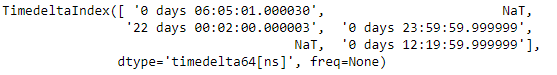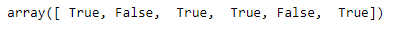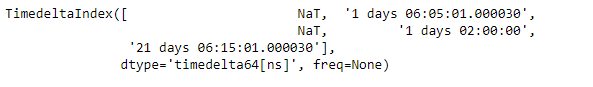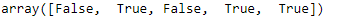# Python | Pandas TimedeltaIndex.notna

Python is a great language for doing data analysis, primarily because of the fantastic ecosystem of data-centric python packages. Pandas is one of those packages and makes importing and analyzing data much easier.

Pandas` TimedeltaIndex.notna()` function detect all the non-missing values from the given TimedeltaIndex object. The functionality of this function is opposite of `TimedeltaIndex.isna()`.

Syntax : TimedeltaIndex.notna()

Parameters : None

Return : a boolean array of whether the values are not NA

Example #1: Use `TimedeltaIndex.notna()` function to detect all the non-missing values from the given TimedeltaIndex object.

 `# importing pandas as pd ` `import` `pandas as pd ` ` `  `# Create the TimedeltaIndex object ` `tidx ``=` `pd.TimedeltaIndex(data ``=``[``'06:05:01.000030'``, ``None``, ``'22 day 2 min 3us 10ns'``, ` `                                   ``'+23:59:59.999999'``, ``None``, ``'+12:19:59.999999'``]) ` ` `  `# Print the TimedeltaIndex object ` `print``(tidx) `

Output :Now we will use the `TimedeltaIndex.notna()` function to detect all the non-missing values from the tidx object.

 `# find all non-missing values ` `tidx.notna() `

Output :As we can see in the output, the `TimedeltaIndex.notna()` function has returned a boolean array which contains `True` value corresponding to the non-missing values and `False` value corresponding to the missing values.

Example #2: Use `TimedeltaIndex.notna()` function to detect all the non-missing values from the given TimedeltaIndex object.

 `# importing pandas as pd ` `import` `pandas as pd ` ` `  `# Create the TimedeltaIndex object ` `tidx ``=` `pd.TimedeltaIndex(data ``=``[``None``, ``'1 days 06:05:01.000030'``, ``None``, ` `                       ``'1 days 02:00:00'``, ``'21 days 06:15:01.000030'``]) ` ` `  `# Print the TimedeltaIndex object ` `print``(tidx) `

Output :Now we will use the `TimedeltaIndex.notna()` function to detect all the non-missing values from the tidx object.

 `# find all non-missing values ` `tidx.notna() `

Output :As we can see in the output, the `TimedeltaIndex.notna()` function has returned a boolean array which contains `True` value corresponding to the non-missing values and `False` value corresponding to the missing values.

My Personal Notes arrow_drop_upCheck out this Author's contributed articles.

If you like GeeksforGeeks and would like to contribute, you can also write an article using contribute.geeksforgeeks.org or mail your article to contribute@geeksforgeeks.org. See your article appearing on the GeeksforGeeks main page and help other Geeks.

Please Improve this article if you find anything incorrect by clicking on the "Improve Article" button below.

Article Tags :

Be the First to upvote.

Please write to us at contribute@geeksforgeeks.org to report any issue with the above content.